# NumPy linalg norm## Introduction to NumPy linalg norm function

This Function is used to calculate the matrix Norm or vector norms. This function also presents inside the NumPy library but is meant for calculating the norms. So it can be used to calculate one of the vector norms, or we can say eight of the matrix norm. Matrix norms are nothing, but we can say it is the extension of vector norm. It can also calculate the infinite vector norms. In this topic, we are going to learn about NumPy linalg norm.

Syntax

Below is the syntax of the linalg norm function as per the python doc. This function takes four parameters as the input, which are described below;

`myNumPy.linalg.norm(x, ord=None, axis=None, keepdims=False)`

we will discuss the input parameters in detail in the coming section. Until we see one practice syntax for a better understanding of the function how to use it while programming;

`myNumPy.linalg.norm(param1, param2, param3, param4)`

### How linalg norm function work in NumPy?

As of now, we know that it is used to calculate one of the infinite vector norms or right of the different matrix norms in python. Also, matrix norms are the extension of the vector norm. Now we will see how we can define a matrix norm function, in general, see below;

e.g., f:Rm×n→R  (General function used to define the matrix norm)

As matrix norm is the extension of vector, hence it has similar properties to vector norm. Now we will discuss all parameters of the norm function in detail to understand how to use them while writing programs in python see below;

This function takes 4 parameters which are the following;

1. First parameter -( x ): This parameter takes input as the array type. We can pass an array variable containing various elements. We can also create a random array by using functions available in the Numpy library.

2. Second param – (axis): As we all know, we have an x-axis and y-axis, and here it meant the same. This parameter is the optional parameter in norm function. If we do not pass any value for this parameter, this function will return us matrix norm or a vector norm. But if we pass an integer value for this parameter, then it will compute the vector norm for the x-axis. The matrix norm is calculated if we pass two tuples as the axis value.

3. Third param – (ord): We have different orders available for this param. This ord full form is  ‘order’ only, and it supports different order as well as the name suggest. We will see the different order in detail, which are as follows;

 0 1 -1 2 -2 None Fro Nuc inf -inf

4. Fourth Param – (keepdims): This parameter is of type Boolean its default value is false. If the value is True, then axes are normed in the left of the result; otherwise of false, then the axes are kept in the result. It represents either the false or true value of the parameter.

Now we will see one example of how to use this library in our code;

This function is available inside the linalg library, which is part of NumPy, but here we have to import two libraries one is Numpy, and the other one is linalg. To use the norm function, import linalg to your program.

• To calculate Matrix: In order to calculate the norm for the matrix, we need to pass 2-tuple in axis as the value. Let’s understand the working below;

```from numpy import linalg as NO import numpy as mynum arr = mynum.arange(4) - 2 print(NO.norm(arr))```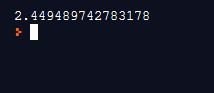In this example, we are calculation the norm for a matrix. In the first line, we are importing the linalg library and NumPy library in order to use their functions.  In the next line, we are creating one array by using the arrange function of the NumPy library. After that, calling the norm function and passing an array as the input parameter. Also, we have defined our alias to use this package name as an alternate short name.

• To calculate the Vector: In order to calculate the norm for a vector, we need to provide the integer value for the axis parameter.

```from numpy import linalg as NO import numpy as mynum arr = mynum.arange(4) - 2 print(NO.norm(arr, axis=0))```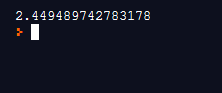In the above example, we are calculating the norm for vector. Here we are passing one more parameter, i.e. axis, which is ‘0’. Apart from this, all things are similar for matrix and vector norm calculation.

Points to remember while working with the Norm function in python:

• In order to use this function, we need to import the linalg library into the program.
• We can calculate the norm for vector and matrix depend upon the logic we have written.

### Examples of NumPy linalg norm

Given below are the examples of NumPy linalg norm:

#### Example #1

In this example, we are calculating the norm for a vector by using the norm function.

Code:

```from numpy import linalg as NO import numpy as mynum # ccreating one array, using nUmpy arrange function arr = mynum.arange(4) - 2 # calculating nirmas for vector here.. print(NO.norm(arr, axis=0))```

Output: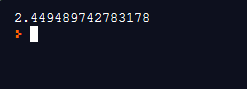#### Example #2

In this example, we are calculating the norm for a matrix by using the norm function. Also, notice we have not mentioned the axis argument here because we are using 2 tuples here.

Code:

```from numpy import linalg as NO import numpy as mynum # ccreating one array, using nUmpy arrange function arr = mynum.arange(4) - 2 # calculating norms for matrix here .. print(NO.norm(arr))```

Output :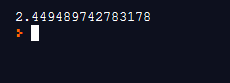#### Example #3

In this example, we are calculating the norm for matrix using norm. Also, using the arrange and reshape function to creating our array.

Code:

```from numpy import linalg as NO import numpy as mynum # array creation here using arrange function arr = mynum.arange(4) - 2 arr = arr.reshape((2,2)) print("array value is  :::") print(arr) #result is after function applied here result  = NO.norm(arr) print("result is  ::") print(result)```

Output: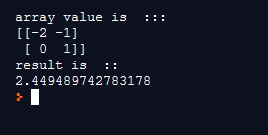### Conclusion

The norm of vector and matrix can be calculated by using the norm function, which is available inside the linalg library. We can calculate the infinite vector norm and eight different matrix norms by using this function. Also, we can pass the reaming parameters as a requirement.

### Recommended Articles

This is a guide to NumPy linalg norm. Here we discuss How the linalg norm function works in NumPy and Examples along with the codes and outputs. You may also have a look at the following articles to learn more –

1. numpy.dot()
2. NumPy Ndarray
3. NumPy Functions
4. numpy.ravel()

The post NumPy linalg norm appeared first on EDUCBA.

This post first appeared on Best Online Training & Video Courses | EduCBA, please read the originial post: here